# Mean State

Period Mean (original grids) [W/m2]
Model Period Mean (intersection) [W/m2]
Model Period Mean (complement) [W/m2]
Benchmark Period Mean (intersection) [W/m2]
Benchmark Period Mean (complement) [W/m2]
Bias [W/m2]
RMSE [W/m2]
Phase Shift [months]
Bias Score 
RMSE Score 
Seasonal Cycle Score 
Spatial Distribution Score 
Interannual Variability Score 
Overall Score 
Benchmark [-] 457.
CRUNCEPv7 [-] 461. 461. 456. 465. 6.21 15.8 1.44 0.455 0.384 0.785 0.917 0.612 0.590
GSWP3v1 [-] 461. 461. 456. 465. 5.39 14.8 1.43 0.498 0.387 0.801 0.995 0.601 0.612
WFDEI [-] 460. 460. 456. 465. 5.42 15.5 1.60 0.517 0.358 0.777 0.976 0.631 0.603
Period Mean (original grids) [W/m2]
Model Period Mean (intersection) [W/m2]
Model Period Mean (complement) [W/m2]
Benchmark Period Mean (intersection) [W/m2]
Benchmark Period Mean (complement) [W/m2]
Bias [W/m2]
RMSE [W/m2]
Phase Shift [months]
Bias Score 
RMSE Score 
Seasonal Cycle Score 
Spatial Distribution Score 
Interannual Variability Score 
Overall Score 
Benchmark [-] 466.
CRUNCEPv7 [-] 481. 481. 467. 458. 14.5 21.8 0.609 0.517 0.502 0.947 1.00 0.622 0.682
GSWP3v1 [-] 478. 478. 467. 458. 11.5 19.5 0.566 0.575 0.514 0.950 0.974 0.613 0.690
WFDEI [-] 476. 476. 467. 458. 9.56 21.3 0.784 0.595 0.456 0.929 0.999 0.663 0.683
Period Mean (original grids) [W/m2]
Model Period Mean (intersection) [W/m2]
Model Period Mean (complement) [W/m2]
Benchmark Period Mean (intersection) [W/m2]
Benchmark Period Mean (complement) [W/m2]
Bias [W/m2]
RMSE [W/m2]
Phase Shift [months]
Bias Score 
RMSE Score 
Seasonal Cycle Score 
Spatial Distribution Score 
Interannual Variability Score 
Overall Score 
Benchmark [-] 443.
CRUNCEPv7 [-] 447. 447. 443. 446. 4.80 16.5 1.06 0.617 0.508 0.868 0.999 0.611 0.685
GSWP3v1 [-] 447. 447. 443. 446. 4.28 15.8 1.12 0.674 0.494 0.854 0.987 0.599 0.684
WFDEI [-] 445. 444. 443. 446. 2.48 17.4 1.50 0.695 0.447 0.794 1.00 0.608 0.665
Period Mean (original grids) [W/m2]
Model Period Mean (intersection) [W/m2]
Model Period Mean (complement) [W/m2]
Benchmark Period Mean (intersection) [W/m2]
Benchmark Period Mean (complement) [W/m2]
Bias [W/m2]
RMSE [W/m2]
Phase Shift [months]
Bias Score 
RMSE Score 
Seasonal Cycle Score 
Spatial Distribution Score 
Interannual Variability Score 
Overall Score 
Benchmark [-] 279.
CRUNCEPv7 [-] 267. 267. 278. 296. -9.37 18.1 0.135 0.854 0.795 0.989 0.995 0.707 0.856
GSWP3v1 [-] 277. 277. 278. 296. 2.04 15.4 0.131 0.907 0.807 0.990 0.999 0.744 0.876
WFDEI [-] 261. 261. 278. 296. -14.9 24.6 0.147 0.794 0.741 0.989 0.999 0.782 0.841
Period Mean (original grids) [W/m2]
Model Period Mean (intersection) [W/m2]
Model Period Mean (complement) [W/m2]
Benchmark Period Mean (intersection) [W/m2]
Benchmark Period Mean (complement) [W/m2]
Bias [W/m2]
RMSE [W/m2]
Phase Shift [months]
Bias Score 
RMSE Score 
Seasonal Cycle Score 
Spatial Distribution Score 
Interannual Variability Score 
Overall Score 
Benchmark [-] 457.
CRUNCEPv7 [-] 455. 455. 454. 467. 2.62 13.7 1.87 0.313 0.388 0.708 0.853 0.524 0.529
GSWP3v1 [-] 457. 457. 454. 467. 3.88 14.0 1.70 0.300 0.387 0.734 0.581 0.565 0.492
WFDEI [-] 459. 459. 454. 467. 6.14 15.1 1.92 0.287 0.359 0.699 0.740 0.654 0.516
Period Mean (original grids) [W/m2]
Model Period Mean (intersection) [W/m2]
Model Period Mean (complement) [W/m2]
Benchmark Period Mean (intersection) [W/m2]
Benchmark Period Mean (complement) [W/m2]
Bias [W/m2]
RMSE [W/m2]
Phase Shift [months]
Bias Score 
RMSE Score 
Seasonal Cycle Score 
Spatial Distribution Score 
Interannual Variability Score 
Overall Score 
Benchmark [-] 312.
CRUNCEPv7 [-] 311. 310. 311. 334. -0.584 9.49 0.0437 0.941 0.887 0.997 0.992 0.854 0.926
GSWP3v1 [-] 316. 316. 311. 334. 5.08 11.3 0.0416 0.914 0.885 0.997 1.00 0.856 0.923
WFDEI [-] 306. 306. 311. 334. -5.31 17.8 0.0327 0.915 0.781 0.998 0.998 0.833 0.884
Period Mean (original grids) [W/m2]
Model Period Mean (intersection) [W/m2]
Model Period Mean (complement) [W/m2]
Benchmark Period Mean (intersection) [W/m2]
Benchmark Period Mean (complement) [W/m2]
Bias [W/m2]
RMSE [W/m2]
Phase Shift [months]
Bias Score 
RMSE Score 
Seasonal Cycle Score 
Spatial Distribution Score 
Interannual Variability Score 
Overall Score 
Benchmark [-] 364.
CRUNCEPv7 [-] 363. 362. 363. 399. 1.27 13.6 0.117 0.864 0.829 0.991 1.00 0.792 0.884
GSWP3v1 [-] 366. 366. 363. 399. 4.67 14.4 0.103 0.848 0.826 0.992 0.993 0.755 0.873
WFDEI [-] 359. 359. 363. 399. -2.13 19.9 0.150 0.833 0.745 0.989 0.998 0.761 0.845
Period Mean (original grids) [W/m2]
Model Period Mean (intersection) [W/m2]
Model Period Mean (complement) [W/m2]
Benchmark Period Mean (intersection) [W/m2]
Benchmark Period Mean (complement) [W/m2]
Bias [W/m2]
RMSE [W/m2]
Phase Shift [months]
Bias Score 
RMSE Score 
Seasonal Cycle Score 
Spatial Distribution Score 
Interannual Variability Score 
Overall Score 
Benchmark [-] 448.
CRUNCEPv7 [-] 455. 456. 446. 467. 11.2 18.9 0.610 0.563 0.586 0.942 0.998 0.654 0.722
GSWP3v1 [-] 451. 451. 446. 467. 7.04 17.1 0.677 0.643 0.573 0.931 0.965 0.621 0.717
WFDEI [-] 452. 452. 446. 467. 8.20 19.9 0.681 0.610 0.516 0.937 0.979 0.643 0.700
Period Mean (original grids) [W/m2]
Model Period Mean (intersection) [W/m2]
Model Period Mean (complement) [W/m2]
Benchmark Period Mean (intersection) [W/m2]
Benchmark Period Mean (complement) [W/m2]
Bias [W/m2]
RMSE [W/m2]
Phase Shift [months]
Bias Score 
RMSE Score 
Seasonal Cycle Score 
Spatial Distribution Score 
Interannual Variability Score 
Overall Score 
Benchmark [-] 281.
CRUNCEPv7 [-] 273. 274. 281. 288. -6.57 15.1 0.0942 0.887 0.798 0.992 0.984 0.770 0.871
GSWP3v1 [-] 283. 283. 281. 288. 3.53 13.2 0.0829 0.921 0.824 0.993 0.999 0.762 0.887
WFDEI [-] 268. 269. 281. 288. -11.6 23.9 0.0929 0.826 0.711 0.993 0.987 0.828 0.843
Period Mean (original grids) [W/m2]
Model Period Mean (intersection) [W/m2]
Model Period Mean (complement) [W/m2]
Benchmark Period Mean (intersection) [W/m2]
Benchmark Period Mean (complement) [W/m2]
Bias [W/m2]
RMSE [W/m2]
Phase Shift [months]
Bias Score 
RMSE Score 
Seasonal Cycle Score 
Spatial Distribution Score 
Interannual Variability Score 
Overall Score 
Benchmark [-] 441.
CRUNCEPv7 [-] 446. 446. 443. 426. 0.518 12.3 0.380 0.824 0.701 0.967 0.990 0.773 0.826
GSWP3v1 [-] 446. 446. 443. 426. 0.648 12.3 0.382 0.835 0.696 0.969 0.998 0.717 0.818
WFDEI [-] 444. 444. 443. 426. -0.660 17.7 0.473 0.795 0.600 0.960 0.987 0.696 0.773
Period Mean (original grids) [W/m2]
Model Period Mean (intersection) [W/m2]
Model Period Mean (complement) [W/m2]
Benchmark Period Mean (intersection) [W/m2]
Benchmark Period Mean (complement) [W/m2]
Bias [W/m2]
RMSE [W/m2]
Phase Shift [months]
Bias Score 
RMSE Score 
Seasonal Cycle Score 
Spatial Distribution Score 
Interannual Variability Score 
Overall Score 
Benchmark [-] 368.
CRUNCEPv7 [-] 363. 363. 366. 389. -0.518 11.6 0.262 0.852 0.777 0.982 0.998 0.792 0.863
GSWP3v1 [-] 364. 364. 366. 389. 1.15 11.6 0.230 0.859 0.776 0.985 0.997 0.774 0.861
WFDEI [-] 355. 355. 366. 389. -7.64 19.2 0.285 0.766 0.653 0.981 0.991 0.815 0.810
Period Mean (original grids) [W/m2]
Model Period Mean (intersection) [W/m2]
Model Period Mean (complement) [W/m2]
Benchmark Period Mean (intersection) [W/m2]
Benchmark Period Mean (complement) [W/m2]
Bias [W/m2]
RMSE [W/m2]
Phase Shift [months]
Bias Score 
RMSE Score 
Seasonal Cycle Score 
Spatial Distribution Score 
Interannual Variability Score 
Overall Score 
Benchmark [-] 399.
CRUNCEPv7 [-] 377. 377. 376. 409. 2.36 15.5 0.507 0.726 0.679 0.944 0.997 0.679 0.784
GSWP3v1 [-] 380. 380. 376. 409. 5.23 15.4 0.494 0.742 0.672 0.944 0.999 0.687 0.786
WFDEI [-] 375. 375. 376. 409. -0.360 18.7 0.603 0.734 0.611 0.929 0.999 0.735 0.770
Period Mean (original grids) [W/m2]
Model Period Mean (intersection) [W/m2]
Model Period Mean (complement) [W/m2]
Benchmark Period Mean (intersection) [W/m2]
Benchmark Period Mean (complement) [W/m2]
Bias [W/m2]
RMSE [W/m2]
Phase Shift [months]
Bias Score 
RMSE Score 
Seasonal Cycle Score 
Spatial Distribution Score 
Interannual Variability Score 
Overall Score 
Benchmark [-] 450.
CRUNCEPv7 [-] 457. 457. 450. 446. 6.88 16.6 0.344 0.806 0.762 0.973 0.994 0.701 0.833
GSWP3v1 [-] 452. 452. 450. 446. 2.34 15.0 0.322 0.844 0.761 0.976 0.999 0.714 0.843
WFDEI [-] 447. 447. 450. 446. -2.71 19.2 0.411 0.826 0.696 0.965 0.999 0.704 0.814
Period Mean (original grids) [W/m2]
Model Period Mean (intersection) [W/m2]
Model Period Mean (complement) [W/m2]
Benchmark Period Mean (intersection) [W/m2]
Benchmark Period Mean (complement) [W/m2]
Bias [W/m2]
RMSE [W/m2]
Phase Shift [months]
Bias Score 
RMSE Score 
Seasonal Cycle Score 
Spatial Distribution Score 
Interannual Variability Score 
Overall Score 
Benchmark [-] 281.
CRUNCEPv7 [-] 278. 278. 281. 293. -3.57 15.7 0.202 0.896 0.790 0.984 0.986 0.704 0.859
GSWP3v1 [-] 287. 287. 281. 293. 6.28 16.8 0.170 0.886 0.791 0.986 1.00 0.764 0.870
WFDEI [-] 273. 273. 281. 293. -8.10 21.9 0.193 0.858 0.721 0.985 0.982 0.829 0.849
Period Mean (original grids) [W/m2]
Model Period Mean (intersection) [W/m2]
Model Period Mean (complement) [W/m2]
Benchmark Period Mean (intersection) [W/m2]
Benchmark Period Mean (complement) [W/m2]
Bias [W/m2]
RMSE [W/m2]
Phase Shift [months]
Bias Score 
RMSE Score 
Seasonal Cycle Score 
Spatial Distribution Score 
Interannual Variability Score 
Overall Score 
Benchmark [-] 442.
CRUNCEPv7 [-] 443. 443. 439. 459. 8.32 16.8 0.816 0.557 0.562 0.896 0.998 0.676 0.709
GSWP3v1 [-] 444. 444. 439. 459. 8.98 16.4 0.854 0.562 0.573 0.894 0.961 0.667 0.705
WFDEI [-] 445. 445. 439. 459. 10.1 19.1 0.916 0.543 0.507 0.887 0.983 0.717 0.691
Period Mean (original grids) [W/m2]
Model Period Mean (intersection) [W/m2]
Model Period Mean (complement) [W/m2]
Benchmark Period Mean (intersection) [W/m2]
Benchmark Period Mean (complement) [W/m2]
Bias [W/m2]
RMSE [W/m2]
Phase Shift [months]
Bias Score 
RMSE Score 
Seasonal Cycle Score 
Spatial Distribution Score 
Interannual Variability Score 
Overall Score 
Benchmark [-] 386.
CRUNCEPv7 [-] 388. 388. 384. 429. 6.21 11.1 0.106 0.860 0.859 0.993 0.988 0.866 0.904
GSWP3v1 [-] 390. 390. 384. 429. 7.99 11.9 0.0700 0.839 0.861 0.995 0.998 0.851 0.901
WFDEI [-] 383. 383. 384. 429. 1.09 16.4 0.0844 0.895 0.730 0.994 0.985 0.901 0.873
Period Mean (original grids) [W/m2]
Model Period Mean (intersection) [W/m2]
Model Period Mean (complement) [W/m2]
Benchmark Period Mean (intersection) [W/m2]
Benchmark Period Mean (complement) [W/m2]
Bias [W/m2]
RMSE [W/m2]
Phase Shift [months]
Bias Score 
RMSE Score 
Seasonal Cycle Score 
Spatial Distribution Score 
Interannual Variability Score 
Overall Score 
Benchmark [-] 324.
CRUNCEPv7 [-] 324. 324. 323. 347. 1.68 10.7 0.0546 0.911 0.844 0.996 0.987 0.781 0.894
GSWP3v1 [-] 329. 329. 323. 347. 6.71 13.5 0.0540 0.872 0.833 0.996 0.966 0.779 0.880
WFDEI [-] 320. 320. 323. 347. -2.43 18.4 0.0791 0.906 0.727 0.994 0.992 0.844 0.865
Period Mean (original grids) [W/m2]
Model Period Mean (intersection) [W/m2]
Model Period Mean (complement) [W/m2]
Benchmark Period Mean (intersection) [W/m2]
Benchmark Period Mean (complement) [W/m2]
Bias [W/m2]
RMSE [W/m2]
Phase Shift [months]
Bias Score 
RMSE Score 
Seasonal Cycle Score 
Spatial Distribution Score 
Interannual Variability Score 
Overall Score 
Benchmark [-] 377.
CRUNCEPv7 [-] 377. 378. 377. 378. 1.16 13.8 0.0965 0.843 0.816 0.993 0.985 0.780 0.872
GSWP3v1 [-] 380. 381. 377. 378. 4.28 13.6 0.0956 0.851 0.817 0.993 1.00 0.730 0.868
WFDEI [-] 376. 377. 377. 378. 0.492 19.3 0.126 0.857 0.713 0.991 0.994 0.815 0.847
Period Mean (original grids) [W/m2]
Model Period Mean (intersection) [W/m2]
Model Period Mean (complement) [W/m2]
Benchmark Period Mean (intersection) [W/m2]
Benchmark Period Mean (complement) [W/m2]
Bias [W/m2]
RMSE [W/m2]
Phase Shift [months]
Bias Score 
RMSE Score 
Seasonal Cycle Score 
Spatial Distribution Score 
Interannual Variability Score 
Overall Score 
Benchmark [-] 449.
CRUNCEPv7 [-] 457. 457. 449. 463. 7.89 14.1 1.11 0.345 0.410 0.853 0.961 0.661 0.607
GSWP3v1 [-] 457. 457. 449. 463. 8.33 14.2 1.22 0.342 0.398 0.837 0.999 0.623 0.600
WFDEI [-] 457. 457. 449. 463. 8.68 15.3 1.76 0.346 0.338 0.739 0.988 0.690 0.573
Period Mean (original grids) [W/m2]
Model Period Mean (intersection) [W/m2]
Model Period Mean (complement) [W/m2]
Benchmark Period Mean (intersection) [W/m2]
Benchmark Period Mean (complement) [W/m2]
Bias [W/m2]
RMSE [W/m2]
Phase Shift [months]
Bias Score 
RMSE Score 
Seasonal Cycle Score 
Spatial Distribution Score 
Interannual Variability Score 
Overall Score 
Benchmark [-] 443.
CRUNCEPv7 [-] 447. 447. 443. 434. 3.42 12.5 0.714 0.735 0.591 0.901 1.00 0.672 0.748
GSWP3v1 [-] 447. 447. 443. 434. 3.23 12.7 0.810 0.756 0.573 0.885 0.999 0.646 0.739
WFDEI [-] 443. 443. 443. 434. -0.558 15.5 1.00 0.755 0.494 0.858 0.999 0.680 0.713
Period Mean (original grids) [W/m2]
Model Period Mean (intersection) [W/m2]
Model Period Mean (complement) [W/m2]
Benchmark Period Mean (intersection) [W/m2]
Benchmark Period Mean (complement) [W/m2]
Bias [W/m2]
RMSE [W/m2]
Phase Shift [months]
Bias Score 
RMSE Score 
Seasonal Cycle Score 
Spatial Distribution Score 
Interannual Variability Score 
Overall Score 
Benchmark [-] 383.
CRUNCEPv7 [-] 383. 383. 381. 396. 2.29 19.9 1.02 0.640 0.566 0.866 0.998 0.630 0.711
GSWP3v1 [-] 388. 387. 381. 396. 6.19 20.3 0.938 0.621 0.567 0.881 0.993 0.620 0.708
WFDEI [-] 389. 389. 381. 396. 8.00 22.6 0.945 0.611 0.513 0.884 0.999 0.667 0.698

# Temporally integrated period mean

BENCHMARK MEAN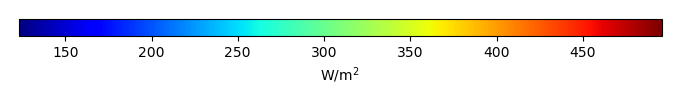MODEL MEANBIAS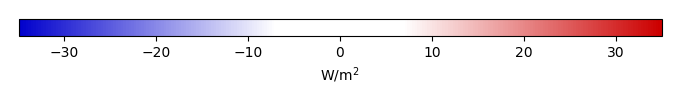BIAS SCORERMSE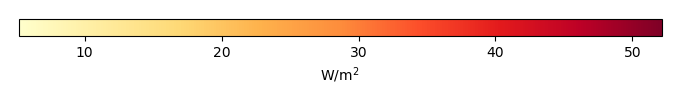RMSE SCOREBENCHMARK INTERANNUAL VARIABILITY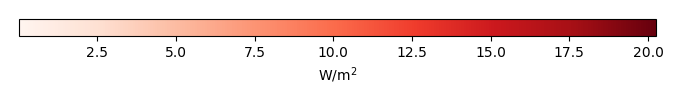MODEL INTERANNUAL VARIABILITYINTERANNUAL VARIABILITY SCOREBENCHMARK MAX MONTHMODEL MAX MONTHDIFFERENCE IN MAX MONTH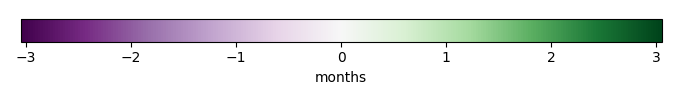SEASONAL CYCLE SCORESPATIAL TAYLOR DIAGRAMMODEL COLORS# Spatially integrated regional mean

MODEL COLORSREGIONAL MEANANNUAL CYCLEMONTHLY ANOMALYANNUAL CYCLE# All Models

BenchmarkCRUNCEPv7GSWP3v1WFDEI# Data Information

creation_date: Thu May 8 23:13:11 PDT 2014

source_file: This product is generated from monthly 1 degree CERES EBAF Radiation observations